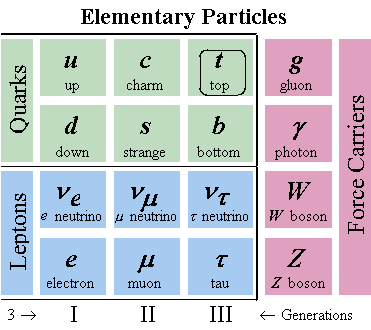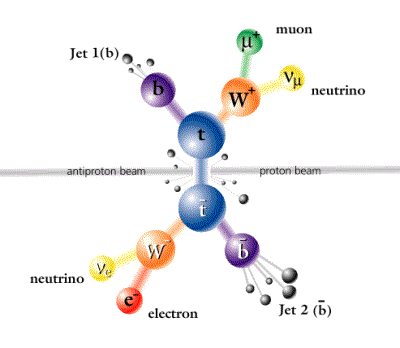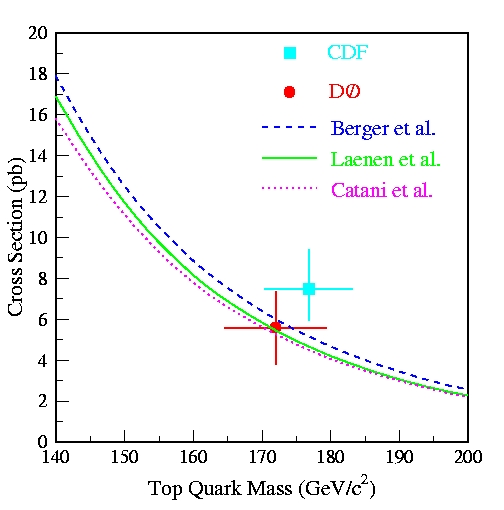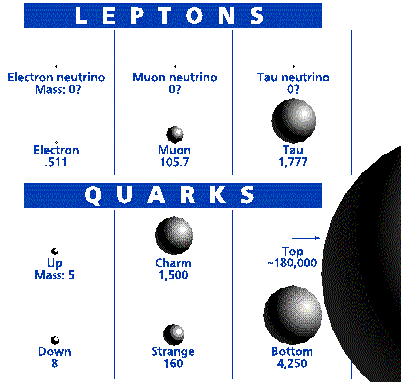# The Top Quark: worth its weight in gold

### Introduction

#### Scientists built the Standard Model of particle physics as a description of the basic nature of the Universe. The top quark is the sixth, and according to the Standard Model, the last to be found. It is one of the fundamental particles of the Standard Model, a "particle zoo" of 6 quarks, 6 leptons, and 4 force-carrying bosons like the photon (the force carrier of light). This Table shows all we currently know:Fig 1. Elementary particles of the Standard Model The missing piece Until a decade ago, almost all particles predicted by this model were found experimentally except for one piece missing, the top quark. The Standard Model requires that quarks (and also leptons) come in pairs. Therefore after the discovery of the b quark in 1977 there was a quark without a partner. All matter as we know it is formed with from the first generation of quarks and leptons (see figure above). Although top quarks are not part of the Universe as we know it today, scientists believe that they existed naturally at the beginning of our Universe, along with other currently non-existent particles. Creating tops After decades of very difficult searches, the top quark was discovered in 1995 by the CDF and DØ experiments at Fermilab. The Fermi National Accelerator Laboratory (Fermilab), located in Batavia, Il, creates the conditions necessary for nature to produce top quarks. During the discovery of the top quark, the Fermilab accelerator, called the Tevatron, collided 900 GeV protons and 900 GeV anti-protons (1 GeV is the kinetic energy that a 1 billion volt battery could give to 1 proton), allowing the creation of top quarks. Anti-protons are like protons but have opposite charge. Now, this accelerator has been updated to collide protons with higher energy, that is 980 GeV protons and 980 GeV anti-protons. More energy means more top quarks ! Not an easy task... CDF and DØ are very large collaborations of more than 500 people. These experiments are complementary of one another, providing cross checks of their results. Top quarks very rarely get produced in proton collisions with anti-protons. Therefore, these experiments consist of very complicated instruments called detectors which use thousands of data readout channels (like pixels on a digital camera) to identify top quarks from other more common processes. ... But predictions helpFig 2: Production and decay of a top quark pair The Standard Model gives scientists guidance on how the top quark will be produced and how it will decay. From the theory, we expect top quarks to be produced predominantly in pairs. The top quark existence is very short. It decays into a lighter mass b quark and a W boson. The existence of the top quark is inferred by the observation of its decay products. Each W boson also decays shortly after being produced, therefore signatures of top pair production depend on the decay of the two W bosons in the event. Most of the times, the W decays into 2 quarks (2/3 of the time) which after a process called "hadronization" appear as a collimated spray or jet of particles in the detector. The rest of the times, the W can decay to one of the three flavors of charged lepton and neutrino. Removing impostors The lepton, neutrino and b quark from the decay of the top quark (and subsequent decay of the W) have some particular kinematic properties. The lepton for example tends to be very energetic compared to leptons coming from other decays. These differences can be used to select which events are top quarks. To study top quarks scientists select candidate events that are similar to what the Standard Model would predict if a top and anti-top quark were produced in the collision. Despite care, this carefully selected sample still contains a large fraction of other types of physics events called background. The major background is when W bosons and jets are created without coming from the top quark together with other light particles. Many techniques are applied to suppress the background further until we consider that we have a cleaner sample of top events. Then we ask two experimental questions: What percent of the time are top quarks produced when we collide 900 GeV protons into 900 GeV antiprotons? What is the mass of the top quarks? These two questions are correlated. The first one refers to the concept of a cross section. A cross section is a description of how often a process will occur under specified conditions. Experimentally the procedure consists of counting the number of times top quarks are seen in our detector and dividing by the total number of collisions observed. The unit measurement of cross sections is the barn (b), defined as 10^-28 m^2, which is about the size of a large atomic nucleus. The bigger the area, the more likely one is to get a collision which will produce the desired outcome. The fact that top quark are very rare, translates into a very small cross section and is therefore measured in picobarns (pb = 10^-12 b), this is a million million times smaller than a large nucleus! One of the main reason why there is a small probability of producing top quarks is because they are very heavy. The energy of the Tevatron accelerator is close to the energy threshold necessary to produce a pair of top quarks.Fig 3. Top quark pair production cross section versus its mass. The CDF and DŘ result for the cross section and mass are shown as a lightblue and red dot with lines corresponding to the uncertainties of our measurement The importance of this cross section measurement is that it gives us a tool to test of the correctness of the Standard Model, so we can compare our experimental result to theoretical calculations. Since the top quark mass is not predicted by the Standard Model (actually the SM doesn't predict any particle mass), the theory allows prediction of the cross section in terms of the top quark's mass. The graph to the right plots the top quark pair production cross section versus its mass (with a cross corresponding to the uncertainties.) The DŘ and CDF results for the cross section and mass are shown as a red dot and lightblue square with lines corresponding to the uncertainties of our measurement. These are the results from data collected previously (Run I of the Tevatron). The figure also shows some lines for various theoretical predictions. We can see that at this time the experimental results are in good agreement with theoretical predictions, within our experimental uncertainties. Why measure the top quark mass ? The top quark is just about as heavy as an atom of gold. It is by far heavier than the other quarks, and is the heaviest particle known to exist.Fig 4. Fermion masses. In fact, the only Standard Model particle it may be lighter than is the Higgs boson. The Higgs boson was input into the Standard Model to account for why the photon does not have mass, but the Z and W bosons do. The Higgs also gives mass to the quarks and leptons, giving more mass to ones that it interacts with the most. The problem is that while the Higgs theoretically does the job it is supposed to do, it has not yet been discovered to actually exist. To help experimenters look for the Higgs boson, theorists have used the Standard Model to predict a relationship between the mass of the top quark and the W boson, which allows one to predict the mass of the Higgs boson. This relationship is extremely sensitive to the top mass, and since the top mass has big errors (as in the plot above), there is still much uncertainty in the predicted Higgs mass. If experimenters knew the predicted mass of the Higgs boson, they would know its cross-section and how it would decay, allowing their searches for the Higgs to be more precise. Therefore, if the top quark mass measurement is improved, the Higgs mass can be more precisely found, and we will be one step closer to answering the question of how particles have mass. Well behaved? There are other properties of the top quark that need to be tested. Does it behave as the Standard model predicts? Its large mass causes scientists to speculate about the role of the top quark in the Standard Model. Being so much more massive than all the other quarks (see figure above), the top quark might play a special part in particle physics. It can also test the Standard Model at energies that have not been studied before, where we may find that our interpretation of the building blocks of nature is not correct or not precise enough. And physicists are always looking for new physics beyond the Standard Model. A new spin? Another test is how the top decays. The Standard Model has specific predictions for the direction that the decay products of the top travel. This is due to the fact that the W boson has a spin which can have several directions relative to its movement. This 'spin' is kind of like the spin on a baseball, but has very specific values. One of these W spins is prohibited by the Standard Model, and the other two spins are predicted to occur a specific fraction of the time. Therefore, if we find that the fractions are wrong, or that the prohibited spin actually occurs, then we know that some physics beyond the Standard Model is happening. And that's what we're looking for :) The top quark is so uniquely heavy, physicists want to make sure that everything about it is exactly as predicted. Also, if you want to find something new, you have to push the energy frontier to the very edge, and currently this means studying the top quark.

Florencia Canelli
University of Rochester, DØ Experiment
canelli@fnal.gov